## 12.1. Integration methods

Differential equations may be solved using several methods that can be selected with the DifferentialEquation.select() method of the differential equation object. Below is the description of each method.

### 12.1.1. Forward Euler

Considering an equation of the form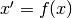,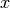is updated according to: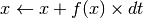### 12.1.2. Runge-Kutta second order

Considering an equation of the form,is updated according to: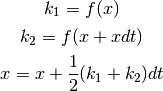### 12.1.3. Runge-Kutta fourth order

Considering an equation of the form,is updated according to: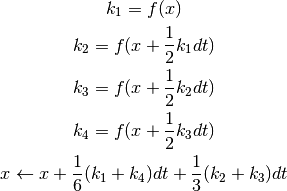### 12.1.4. Exponential Euler

Considering an equation of the form,is updated according to: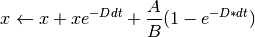## 12.2. Asynchronicity

Most computational paradigms linked to artificial neural networks (using rate code) or cellular automata use implicitly what is called synchronous evaluation of activity. This means that information at time t+dt is evaluated exclusively on information available at time t. The explicit numerical procedure to perform such a synchronized update is to implement a temporary buffer at the unit level where activity computed at time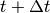is stored. Once all units have evaluated their activity at time, The current activity is replaced by the content of the buffer. We point out that other update procedures have been developed [Lambert:1991] but the basic idea remains the same, namely not to mix information between timeand time. To perform such a synchronization, there is thus a need for a global signal that basically tell units that evaluation is over and they can replace their previous activity with the newly computed one. At the computational level, this synchronization is rather expensive and is mostly justified by the difficulty of handling asynchronous models.

For example, cellular automata have been extensively studied during the past decades for the synchronous case and many theorems has been proved in this context. However, some recent works on asynchronous cellular automata showed that the behavior of these same models and associated properties may be of a radical different nature depending on the level of synchrony of the model (you can asynchronously evaluate only a subpart of all the available automata). In the framework of computational neuroscience we may then wonder what is the relevance of synchronous evaluation since most generally, the system of equations is supposed to give account of a population of neurons that have no reason to be synchronized (if they are not provided with an explicit synchronization signal).

In [Taouali:2009] and [Rougier:2010], we’ve been studying the effect of such asynchronous computation (uniform or non-uniform) on neural networks and more specifically for the case of dynamic neural fields. The whole story is that if you choose a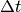small enough, asynchronous and synchronous computation may be considered to lead tp the same result provided the leak term in your equation is not too strong. This is reason why currently, the asynchronous part of dana has been disabled.